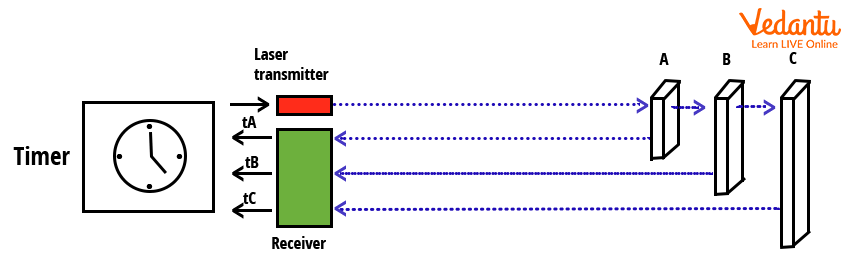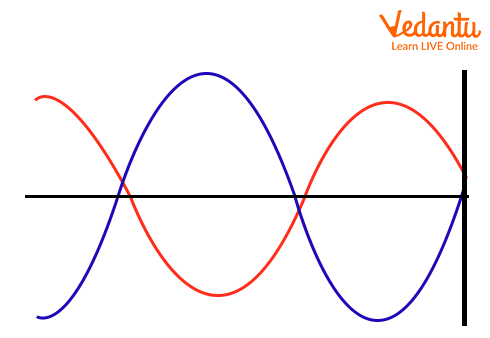Courses
Courses for Kids
Free study material
Free LIVE classes
More

# Time of Flight for JEELIVE
Join Vedantu’s FREE Mastercalss

## An Overview of Time of Flight

The concept of the time of flight depicts the measurement of the time taken by the object, wave, and particles for travel distance through the medium. With the help of the time of flight, knowledge about the medium properties and particle’s nature can be gained. The time of flight is used to measure the velocity of the particles or path length of the particle for understanding the behaviour of particles in the medium. The determination of the time of flight of the travelling body in the medium can be evaluated by the direct time of flight or indirect time of flight.

This article gives a clarity on the concept of time of flight. It discusses a few sections such as the time of flight sensor, derivation of time of flight, principles of flight, time of flight cameras, working of the principle of sensor, and so on.

## Define Time of Flight

The time of flight is a method for determining the distance between the sensor and an object by considering the difference in time between the emission sensor and its return after getting reflected from the object back to the sensor. For the determination of the following aspect, waves of light and sound are commonly used. It depends upon the technology different types of waves with different wavelengths can be used for the same. The Sensors used for the determination of the distance between the source and the object with the help of the time of flight concept are known as the Time of flight sensors.  This concept works on the principle of time of flight principle.

## Principle of Time of Flight Sensors

The principle of time of flight sensors depicts the measurement of the time taken by the wave for travel from the source to the object and return to the source. By using the concept of Physics and Mathematics, the distance between the source and object is evaluated.

Time of flight is one of the techniques used for capturing the images of various dimensions (like 3D images), used in stereoscopic cameras, structured light imaging, etc.

## Determination of the Calculation of Time of Flight Sensors

The determination of time travelled by the wave of light after the emission from the source to the object and getting reflected by the sensors by the simple concept of speed.

The formula which is used for the determination of the time taken by the wave is given by,

• $\text{Speed}=\dfrac{\text{Distance}}{\text{time}}$

According to the concept of time of flight, the wave of light travels from the source to the object and gets back to the source. Therefore, the total distance travelled by the wave will be two times the normal distance between the source and object.

• Distance = 2D

If the wave of light is used in the concept of time of flight, then speed will be the speed of light. Therefore, the speed of light will be denoted as “S”. Hence, the Time taken by the wave of light “T” is given as,

• $\text{Time}=\dfrac{2D}{S}$

The above derivation clarifies the queries like how to calculate the time of flight of the sensors. To determine the distance between the source and object, we will use the following formula:

• $D=\dfrac{S\times T}{2}$

## Working of Time of Flight Sensors

The working of time of flight sensors involves a tiny laser to be fired at the object and it returns after getting reflected from the sensors. Based on the time taken by the wave for travelling from the source to the object and back to the source, we find out the distance between the source and the object. The determination of the distance between the source and object with the help of time taken by the wave. It can be determined by two methods:

• Using pulses of fixed time

• Using shift in phase of an amplitude-modulated wave

In the first method of using pulses of the fixed time, the object is targeted by the wave of light that was transmitted by the laser transmitter, and the time taken by the wave for returning to the receiver of the sensor is measured by the scanner. The pulses sent by the laser transmitter are recorded by the timer. After the evaluation of time, the distance between the sensors and the object is measured. Time of flight cameras are installed alongside the sensors for determining the exact distance between the source and the object.  This method is used to determine the distance between the object and sensors accurately.Schematic Representation of Timer Pulsed Time of Flight Sensor

In the second method of using the phase shift of an amplitude modulated wave, the distance between the source and object is determined by the detection of the phase-shift of the reflected light.Phase Shift of Amplitude Modulated Wave in Time of Flight Sensor

After the modulation of the amplitude, the light of the wave will become a sinusoidal wave with a predetermined frequency.  After that, the detector can easily determine the phase shift of the reflected wave. The formula which is used for the same is given by,

$c=\lambda \times f$

Where,

c = Speed of light

$\lambda=$Wavelength of wave

f = Frequency.

## Conclusion

This article gives information about the concept of time of flight sensors. It discusses the determination of the time of flight of the wave for the determination of the distance between the source and object. It starts with the clarification of the first query, i.e. Define time of flight, how to calculate the time of flight, etc, then it explains the derivation of time of flight, time of flight sensors, and the working principle of the sensor. It covers the major section of the time of flight from a diagram point of view for better clarity of concept.

Last updated date: 24th Sep 2023
Total views: 136.2k
Views today: 3.36k

## FAQs on Time of Flight for JEE

1.  What is the significance of the time of flight monitoring (or ToF monitoring)?

ToF monitoring is used to monitor the working of the time of flight sensors for recording the distance between the source and object. The time of flight monitoring becomes the most important section for analysing the function of each component (like emitter, processor, and receiver) and obtains the best performance of the time of flight sensors as an output. The time of flight monitoring decides the accurate and precise distance between the emitter and the object. Hence, the monitoring of the time of flight sensors is important.

2. What is the working of the indirect time of flight?

The working of the indirect time of flight sensors is to determine the distance between the object and the pixel of the camera. This only works when the object is fully illuminated by the help of the flash of light and evaluates the difference in phase between the emitted light from the flash and the reflection of light from the object. In this way, the indirect time of flight sensors works the determination of the distance between the phase difference between the pixel and object.

3. What is the weightage of this topic in the JEE exam?

The weightage of the topic Time of flight sensors is 3%. This section belongs to the chapter on electromagnetic waves, and wave optics which was found to be an important topic for the JEE mains as well as JEE advance. The expectation of the number of questions was nearly 2-3 questions in the given competitive examination of JEE. In this chapter, it is required to keep a check on every wave equation, and numerical related wave optics and practise a maximum number of questions for better performance in this section.